# 音频D类功放LC滤波器设计（二）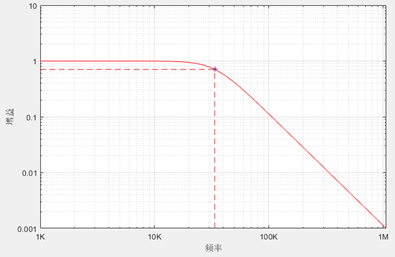LC滤波器的Q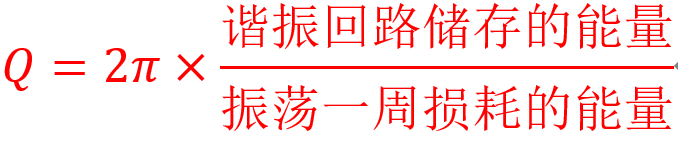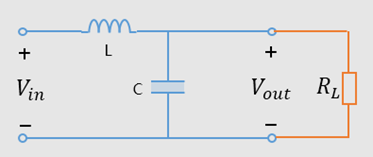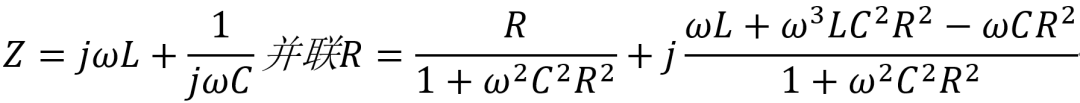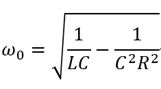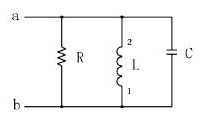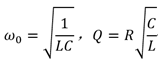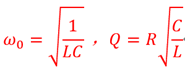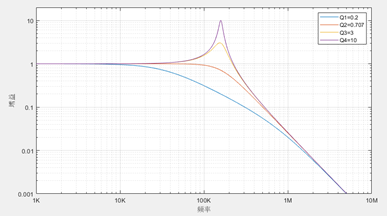CLASS的常用的LC滤波器电路如下图所示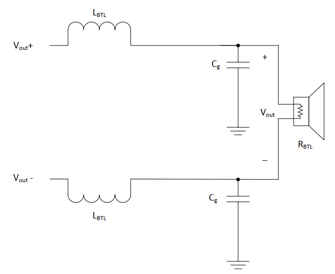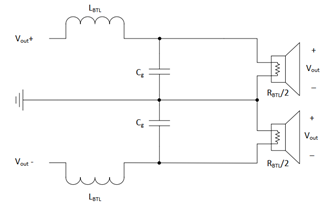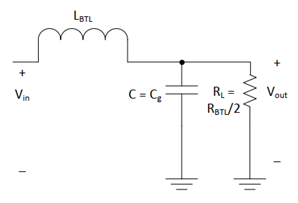LC滤波器设计举例

1、滤波器为LC滤波器，需要确定L，C的值。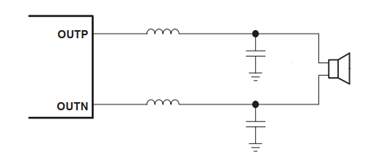2、确定滤波器截止频率，由前面可知，截止频率设计在f=30Khz比较合理。

3、根据差分的等效电路，LC滤波器等效电路如下，R=8Ω/2=4Ω4、我们列出已知量和等式：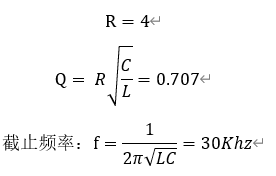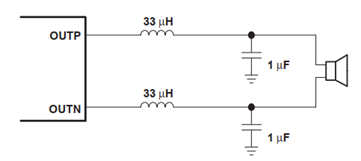``````f=[1000:100:100000000];   %频率范围1Khz-10Mhz
w=(f.*pi*2);              %角频率

C=0.000001;    %1uF   电容量
L=0.000033;    %33uH   电感量
R=4;          %等效负载（可能是喇叭阻抗的一般，根据情况而定）

Zc=1./(w.*C.*1i);   %电容阻抗
Zl=w.*L.*1i;        %电感阻抗

Q=R*((C/L)^0.5);  %滤波器Q值
Av=abs(((Zc.*R)./(Zc+R))./(((Zc.*R)./(Zc+R))+Zl)); %负载1对应增益

figure;       %画图
loglog(f,Av);
legend(['Q=',num2str(Q)]);%Q值
grid on;      %显示网格
set(gca,'YLim',[0.001 20]);%y轴的数据显示范围
set(gca, 'XTickLabel' ,{'1K','10K','100K','1M','10M','100M'}); %x轴频率数据
set(gca, 'YTickLabel' ,{'0.001','0.01','0.1','1','10','100','1000'});   %x轴幅度数据
xlabel('频率'), ylabel('增益');  %x，y轴名称``````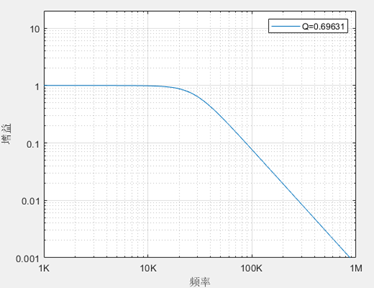5、确定电感的额定电流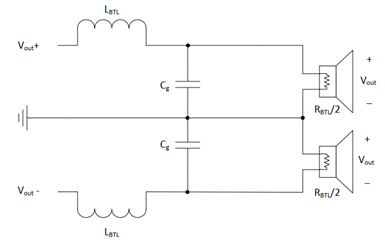0/200